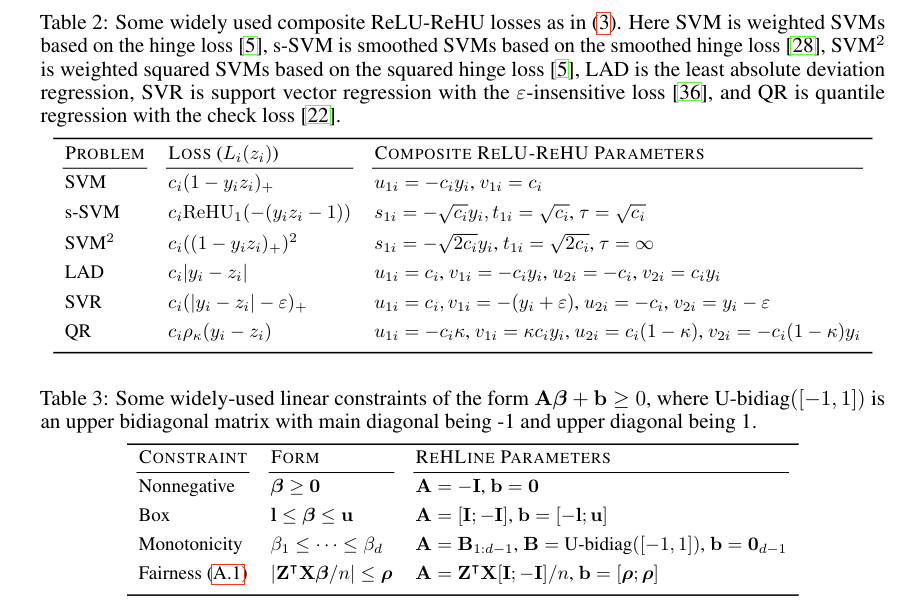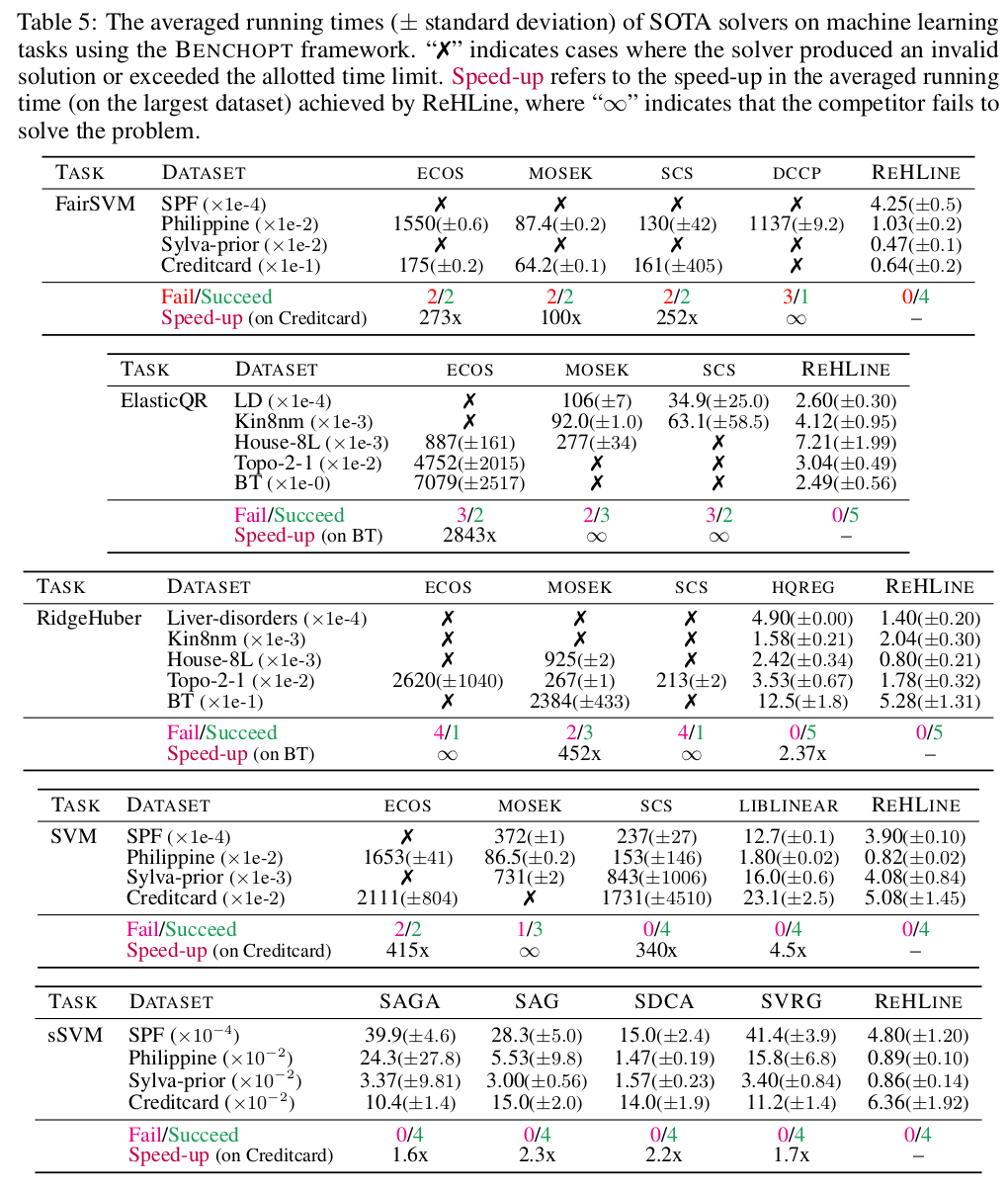# 🦌 ReHLine

ReHLine is designed to be a computationally efficient and practically useful software package for large-scale ERMs.

ReHLine is designed to be a computationally efficient and practically useful software package for large-scale ERMs.

The proposed ReHLine solver has four appealing linear properties:

• It applies to any convex piecewise linear-quadratic loss function, including the hinge loss, the check loss, the Huber loss, etc.

• In addition, it supports linear equality and inequality constraints on the parameter vector.

• The optimization algorithm has a provable linear convergence rate.

• The per-iteration computational complexity is linear in the sample size.

# 🔨 Installation

Install rehline using pip

pip install rehline


See more details in installation.

## 📮 Formulation

ReHLine is designed to address the empirical regularized ReLU-ReHU minimization problem, named ReHLine optimization, of the following form:

$\min_{\mathbf{\beta} \in \mathbb{R}^d} \sum_{i=1}^n \sum_{l=1}^L \text{ReLU}( u_{li} \mathbf{x}_i^\intercal \mathbf{\beta} + v_{li}) + \sum_{i=1}^n \sum_{h=1}^H {\text{ReHU}}_{\tau_{hi}}( s_{hi} \mathbf{x}_i^\intercal \mathbf{\beta} + t_{hi}) + \frac{1}{2} \| \mathbf{\beta} \|_2^2, \ \text{ s.t. } \mathbf{A} \mathbf{\beta} + \mathbf{b} \geq \mathbf{0},$

where $$\mathbf{U} = (u_{li}),\mathbf{V} = (v_{li}) \in \mathbb{R}^{L \times n}$$ and $$\mathbf{S} = (s_{hi}),\mathbf{T} = (t_{hi}),\mathbf{\tau} = (\tau_{hi}) \in \mathbb{R}^{H \times n}$$ are the ReLU-ReHU loss parameters, and $$(\mathbf{A},\mathbf{b})$$ are the constraint parameters. This formulation has a wide range of applications spanning various fields, including statistics, machine learning, computational biology, and social studies. Some popular examples include SVMs with fairness constraints (FairSVM), elastic net regularized quantile regression (ElasticQR), and ridge regularized Huber minimization (RidgeHuber).To generate benchmark results in our paper, please check ReHLine-benchmark.

Problem

Results

FairSVM

Result

ElasticQR

Result

RidgeHuber

Result

SVM

Result

sSVM

Result

Note: You may select the “log-log scale” option in the left sidebar, as this will significantly improve the readability of the results.

## 🧾 Overview of Results## Reference

If you use this code please star the repository and cite the following paper:

@article{daiqiu2023rehline,
title={ReHLine: Regularized Composite ReLU-ReHU Loss Minimization with Linear Computation and Linear Convergence},
author={Dai, Ben and Yixuan Qiu},
journal={Advances in Neural Information Processing Systems},
year={2023},
}